# Chapter 4 Datasets

Below are two public (open-access) and real datasets that we will use for the analyses. For each dataset, you will find its description, minor data wrangling (or data manipulation), and descriptive statistics in both numeric and visual formats.

## 4.1 Salaries Dataset

datasetSalaries <- carData::Salaries

One of the datasets that we’ll use is the Salaries dataset within the carData package. The dataset consists of nine-month salaries collected from 397 collegiate professors in the U.S. during 2008 to 2009. In addition to salaries, the professor’s rank, sex, discipline, years since Ph.D., and years of service was also collected. Thus, there is a total of 6 variables, which are described below.

Variable Variable Type Description
rank Categorical Professor’s rank of either assistant professor, associate professor, or professor
discipline Categorical Type of department the professor works in, either applied or theoretical
yrs.since.phd Continuous Number of years since the professor has obtained their PhD
yrs.service Continuous Number of years the professor has served the department and/or university
sex Categorical Professor’s sex of either male or female
salary Continuous Professor’s nine-month salary (USD)

### 4.1.1 Data Wrangling

Before running these analyses within the GLM context, let’s clean up the dataset so that it’s readable for us rather than computers.

# spell out rank variables
# rename discipline variables to its meaningful name
# ensure both rank and discipline are factors
datasetSalaries <- datasetSalaries %>%
mutate(
rank = case_when(
rank == "AssocProf" ~ "Associate Professor",
rank == "AsstProf" ~ "Assistant Professor",
rank == "Prof" ~ "Professor"
),
discipline = case_when(
discipline == "A" ~ "Theoretical",
discipline == "B" ~ "Applied"
)
) %>%
mutate(
rank = as.factor(rank),
discipline = as.factor(discipline)
)

### 4.1.2 Descriptive Statistics

It’s also always a good idea to examine the data numerically and visually. Let’s first look at the categorical variables then the continuous variables.

#### 4.1.2.1 Categorical Variables

summary(datasetSalaries$rank) ## Assistant Professor Associate Professor Professor ## 67 64 266 To visualize our data, we will be using the function ggplot(). We won’t be going into detail about plotting since data visualization is out of the book’s scope. However, if interested, check out Grolemund’s and Wickham’s data visualization chapter to learn more about ggplot(). ggplot(data = datasetSalaries, mapping = aes(x = rank)) + geom_bar(aes(y = stat(count) / sum(stat(count))), color = "black", fill = "#3182bd") + theme_classic() + labs(y = "Proportion", x = "", title = "rank")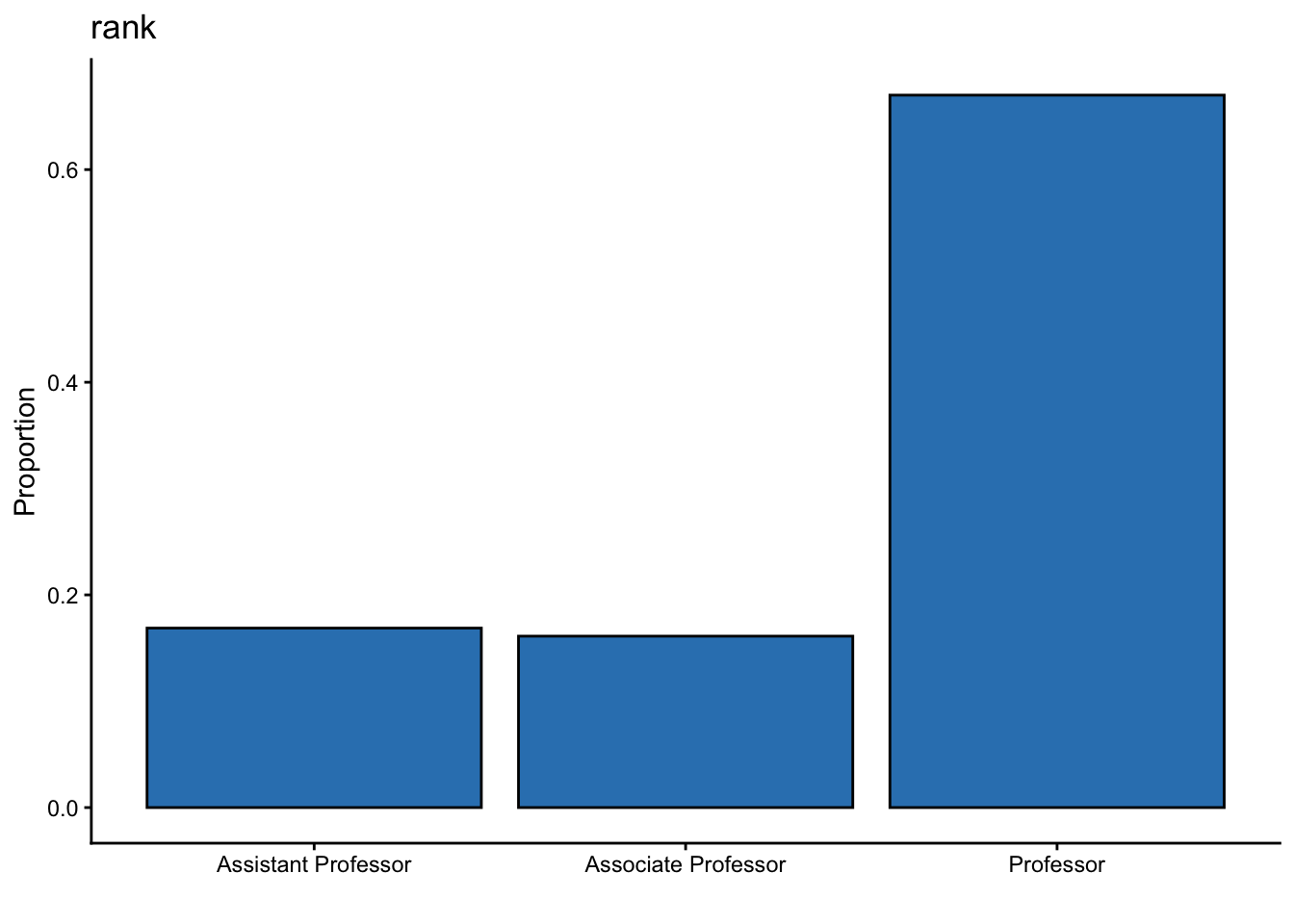In this dataset, there are a lot more professors than assistant and associate professors combined. summary(datasetSalaries$discipline)
##     Applied Theoretical
##         216         181
ggplot(data = datasetSalaries, mapping = aes(x = discipline)) +
geom_bar(aes(y = stat(count) / sum(stat(count))), color = "black", fill = "#3182bd") +
theme_classic() +
labs(y = "Proportion", x = "", title = "discipline")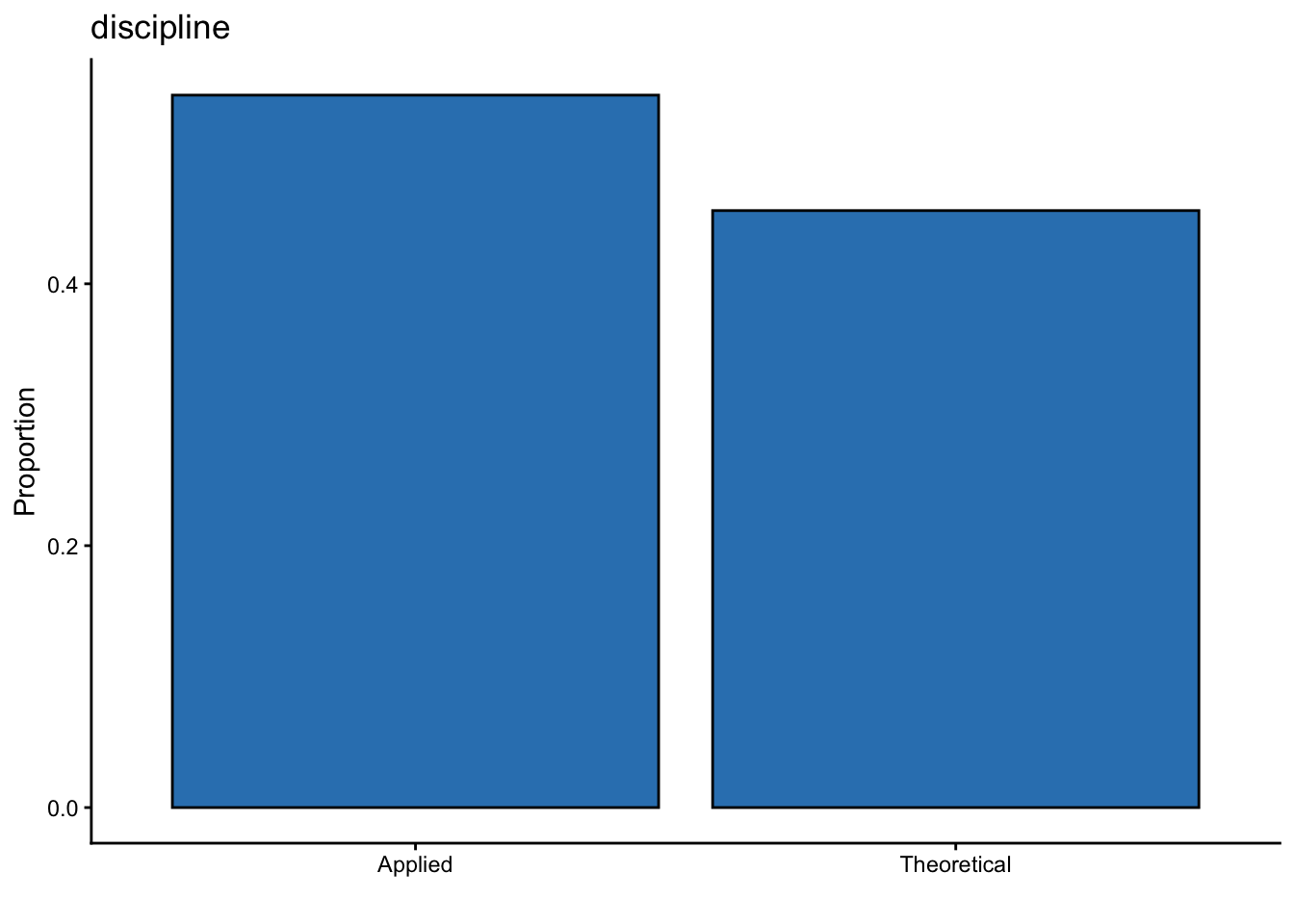There are slightly more professors within the applied than the theoretical discipline (i.e., 35 more).

## 4.2 Anorexia Dataset

datasetAnorexia <- MASS::anorexia

Another dataset that we’ll use is the anorexia dataset within the MASS package. The dataset consists of the weight (in lbs.) of 72 female patients with anorexia before and after either cognitive behavioral therapy, family therapy, or no therapy (control condition).

Variable Variable Type Description
Treatment Categorical Treatment of female patient with anorexia, either cognitive behavioral therapy (CBT), family therapy (FT), or no therepy (CONT)
PreWeight Continuous Weight of female patient with anorexia before treatment in lbs.
PostWeight Continuous Weight of female patient with anorexia after treatment in lbs.

### 4.2.1 Data Wrangling

Again, we can make the dataset slightly more readable for us.

# spell out variable names
# re-order levels to be CBT, FT, then Cont rather than alphabetical order
datasetAnorexia <- datasetAnorexia %>%
mutate(
Treatment = Treat,
PreWeight = Prewt,
PostWeight = Postwt,
Treatment = case_when(
Treatment == "Cont" ~ "Control",
TRUE ~ as.character(Treatment)
),
Treatment = factor(Treatment, levels = c("CBT", "FT", "Control"))
)

### 4.2.2 Descriptive Statistics

#### 4.2.2.1 Categorical Variables

summary(datasetAnorexia\$Treatment)
##     CBT      FT Control
##      29      17      26
ggplot(data = datasetAnorexia, mapping = aes(x = Treatment)) +
geom_bar(aes(y = stat(count) / sum(stat(count))), color = "black", fill = "#3182bd") +
theme_classic() +
labs(y = "Proportion", x = "", title = "Treatment")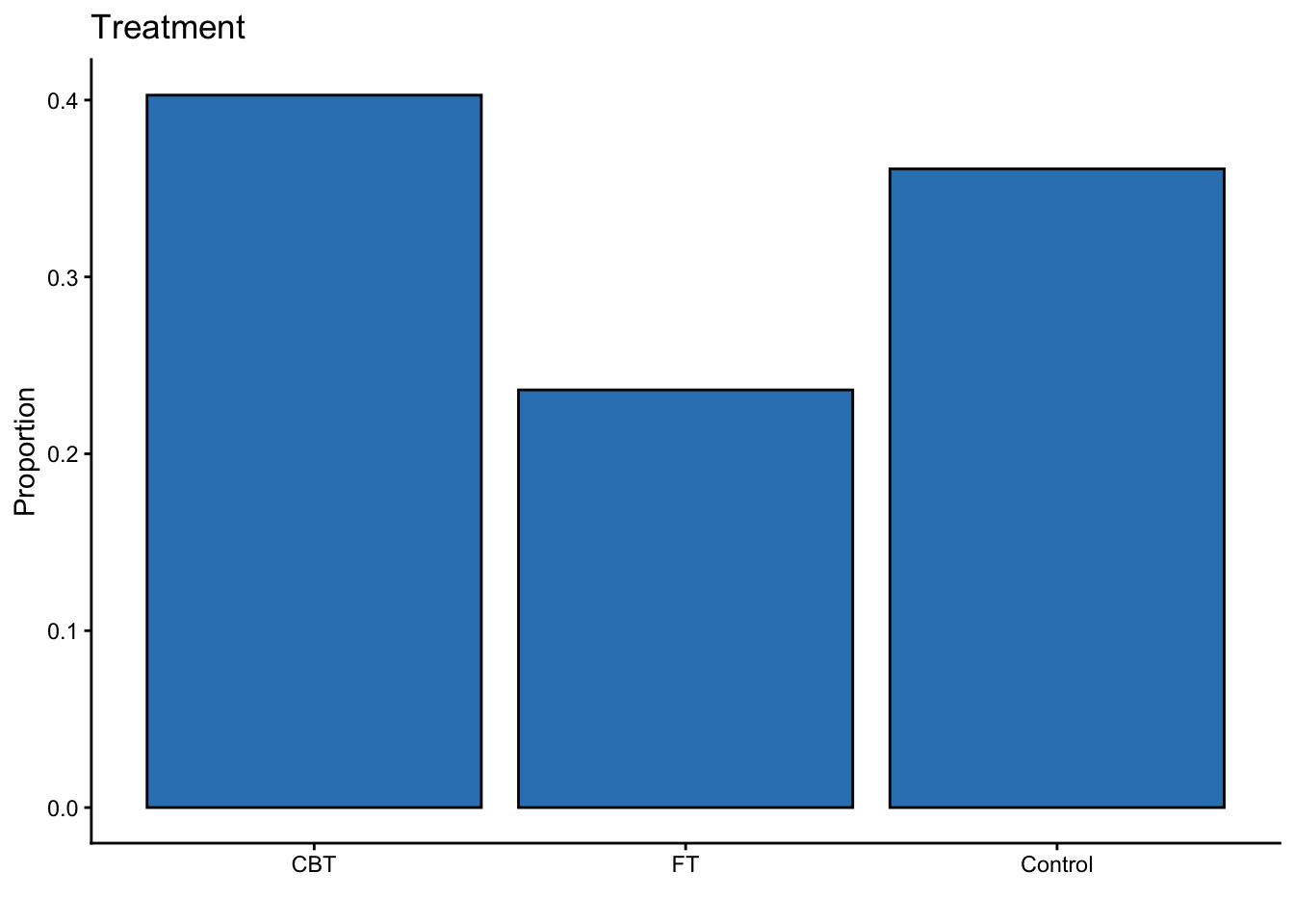There are more participants within the CBT condition compared to either the FT condition or control group.

#### 4.2.2.2 Continuous Variables

datasetAnorexia %>%
select(PreWeight, PostWeight) %>%
describe(.) %>%
round(., 2)
##             n  mean   sd median  min   max  skew kurtosis   se
## PreWeight  72 82.41 5.18  82.30 70.0  94.9 -0.05    -0.16 0.61
## PostWeight 72 85.17 8.04  84.05 71.3 103.6  0.36    -0.81 0.95
ggplot(data = datasetAnorexia, mapping = aes(x = PreWeight)) +
geom_vline(
xintercept = describe(datasetAnorexia)["PreWeight", "mean"],
alpha = .5,
linetype = "dashed"
) +
geom_dotplot(binwidth = 1, fill = "#3182bd") +
geom_text(aes(
x = describe(datasetAnorexia)["PreWeight", "mean"],
label = paste("M =", round(describe(datasetAnorexia)["PreWeight", "mean"], 2)),
y = -.05
),
angle = 0
) +
theme_classic() +
scale_y_continuous(NULL, breaks = NULL) +
labs(x = "", y = "Frequency", title = "Weight Before Study (lbs)")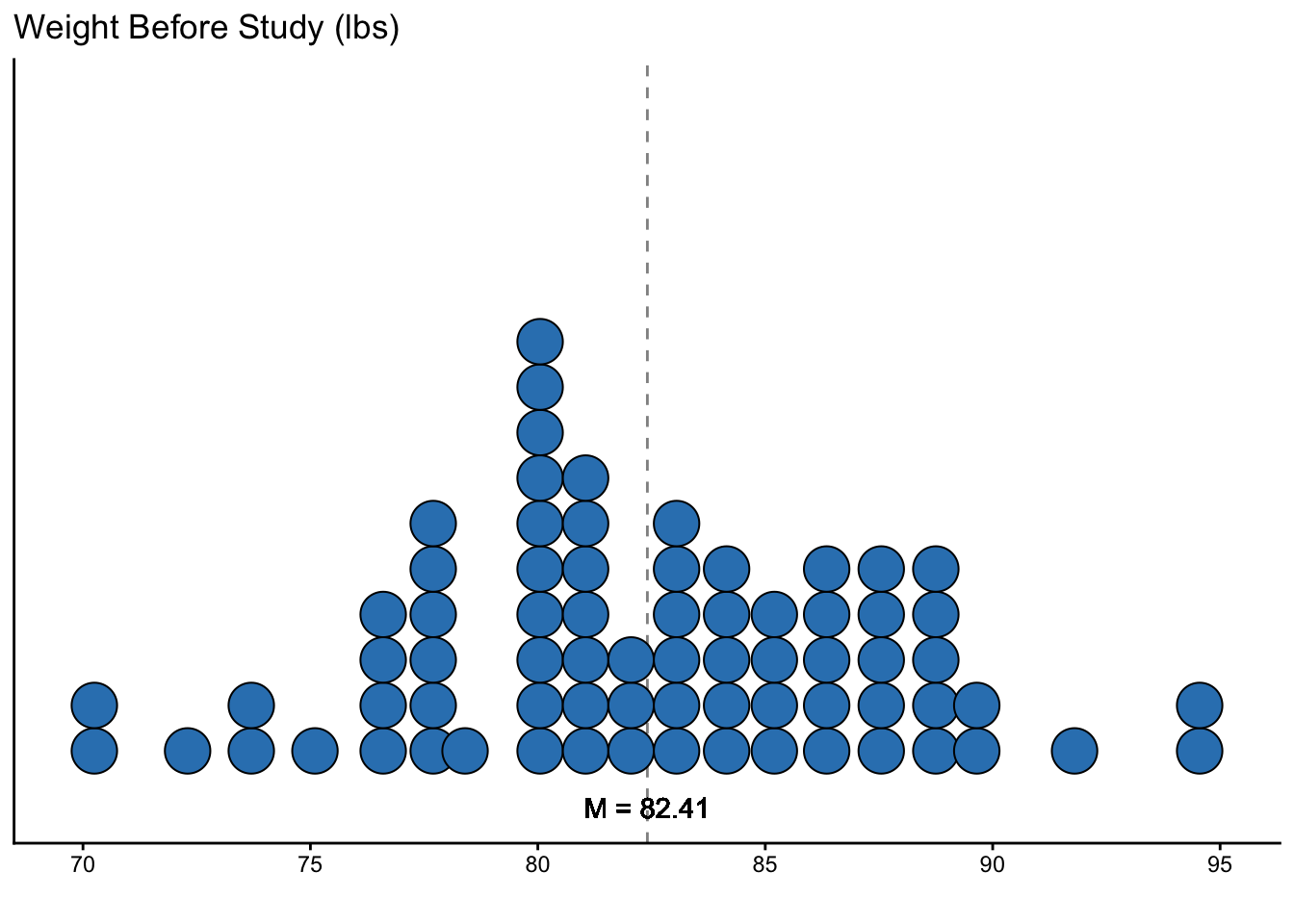On average, women with anorexia before treatment weighed 82.41 lbs.

ggplot(data = datasetAnorexia, mapping = aes(x = PostWeight)) +
geom_vline(
xintercept = describe(datasetAnorexia)["PostWeight", "mean"],
alpha = .5,
linetype = "dashed"
) +
geom_dotplot(binwidth = 1, fill = "#3182bd") +
geom_text(aes(
x = describe(datasetAnorexia)["PostWeight", "mean"],
label = paste("M =", round(describe(datasetAnorexia)["PostWeight", "mean"], 2)),
y = -.05
),
angle = 0
) +
theme_classic() +
scale_y_continuous(NULL, breaks = NULL) +
labs(x = "", y = "Frequency", title = "Weight After Study (lbs)")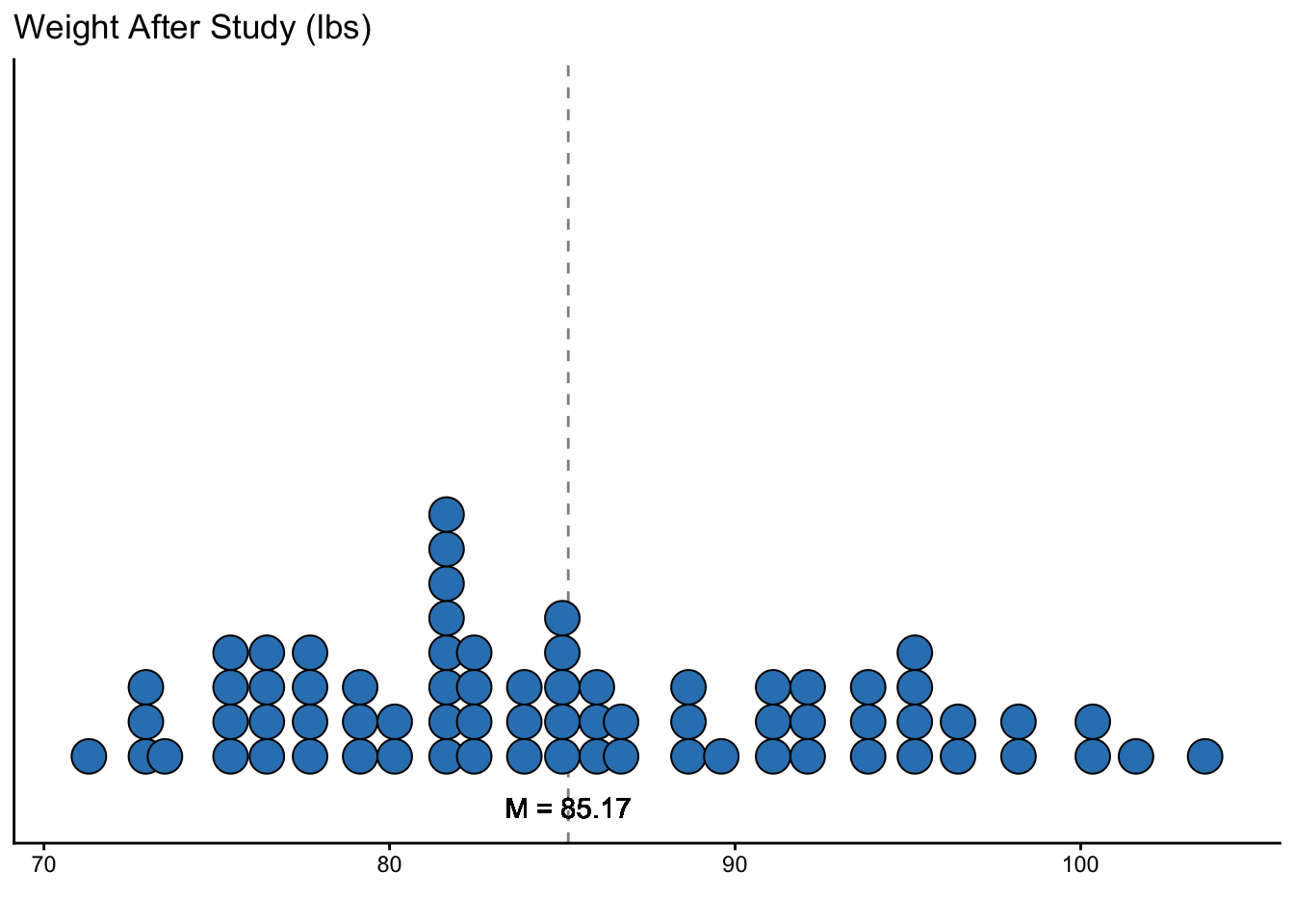On average, women with anorexia after treatment weighed 85.17 lbs, which is about a 2.76 lbs weight gain compared to before treatment.

## 4.3 Use Your Own Dataset

However, if you have an interesting dataset of your own, we encourage you to also try using that dataset alongside ours.